Get instant live expert help with Excel or Google Sheets“My Excelchat expert helped me in less than 20 minutes, saving me what would have been 5 hours of work!”

#### Post your problem and you’ll get Expert help in seconds.

Your message must be at least 40 characters
Our professional Expert are available now. Your privacy is guaranteed.

# How to Count and Sum Colored Cells in Excel

We can count colored cells in excel by using a wide range of functions. In this tutorial, we will learn how to count cells by color and get the sum of colored cells either manually or by doing so across the entire workbook.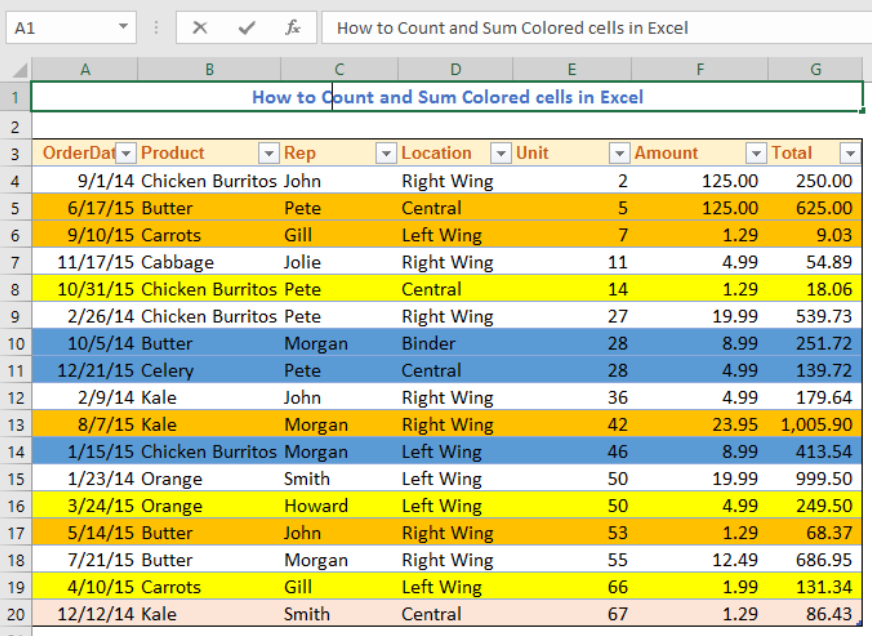Figure 1 – How to count highlighted cells

## How to Count Colored Cells Using Filter and SUBTOTAL

This method counts colored cells without VBA 350 0.  To do this:

• We will insert the formula below into Cell G22

`=SUBTOTAL(102,G1:G20)`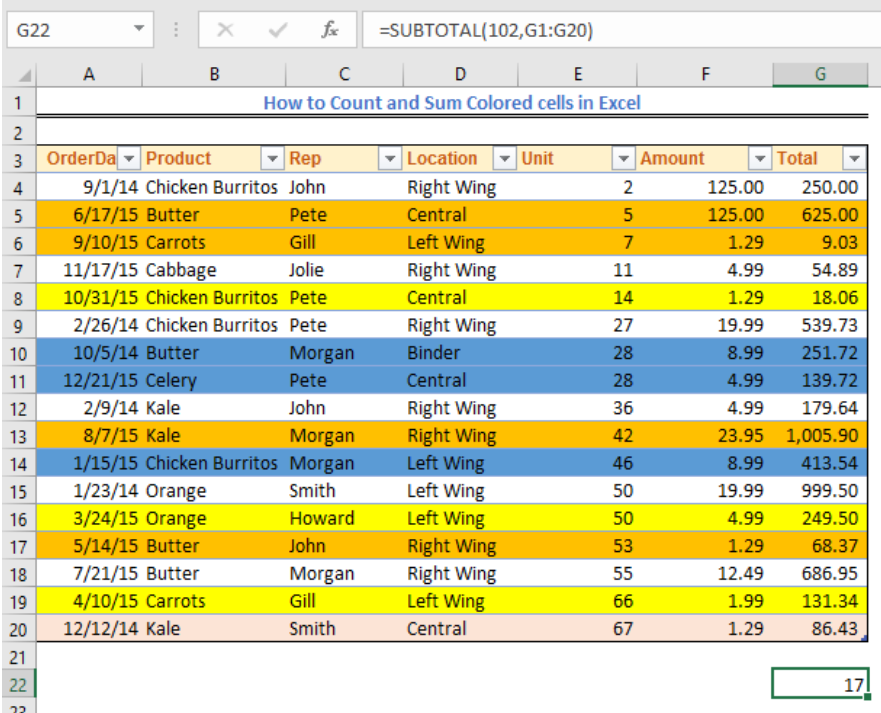Figure 2 – Count colored cells without vba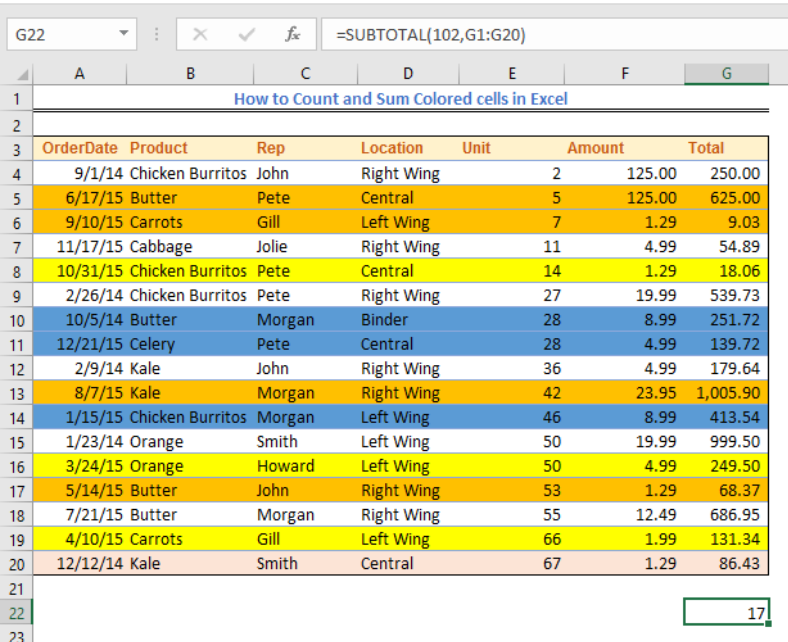Figure 3 – Count colors

• Now we will select the headers
• Next, we will go to Data, select Sort and Filter and lastly filter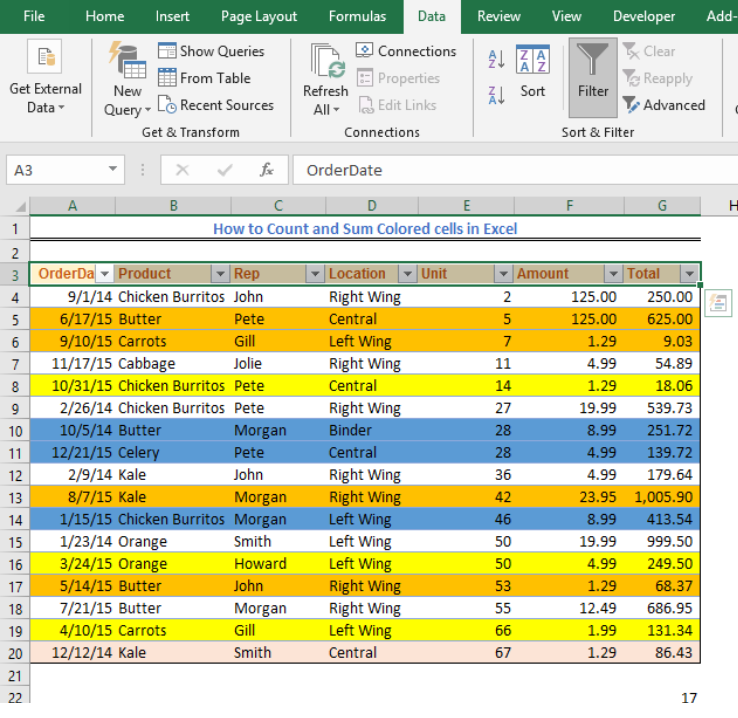Figure 4 – How to count colors

• We will click on any of the filter drop-downs, go to filter by color and select color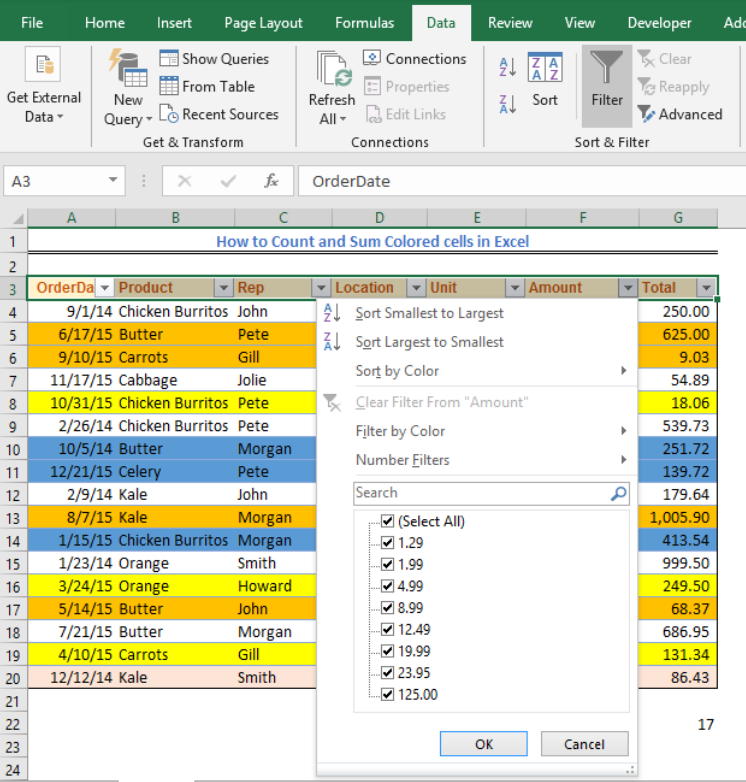Figure 5 – How to count number in colored cells

• Because we have three colors, the filter will show five cells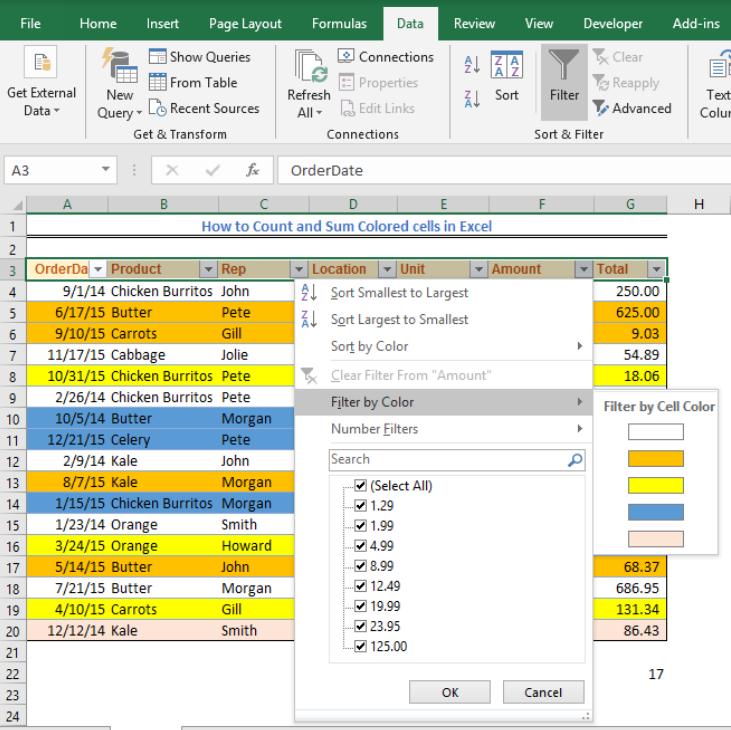Figure 6 – Count colors

• When we filter the cells, we will notice that the value of the SUBTOTAL will change, and we will view only the cells made visible by our formula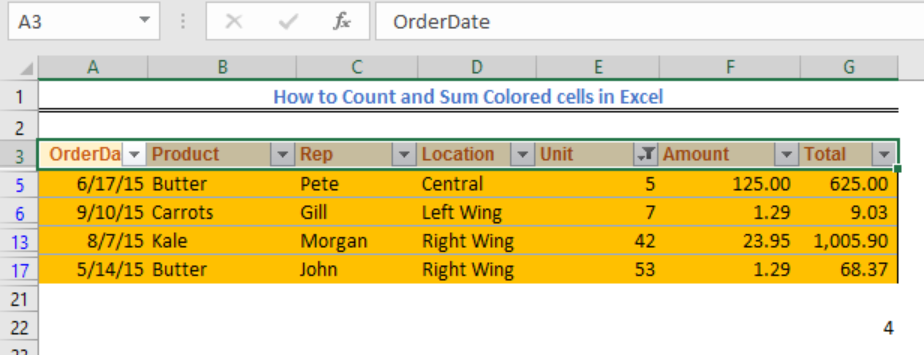Figure 7 – Using SUBTOTAL to count colors

## How to Count Colored Cells Using Countif and GET.CELL Function

The GET.CELL function is a Macro4 function which means we can’t use it directly but must use these steps:

• We will go to formula and select Define Name
• In the New Name dialog, we will enter the following:
• Name: ColorCount
• Scope: Workbook
• Refers to: `=GET.CELL(38,Sheet1!G4)`

Where Sheet 1!G4 is the cell that represents the background color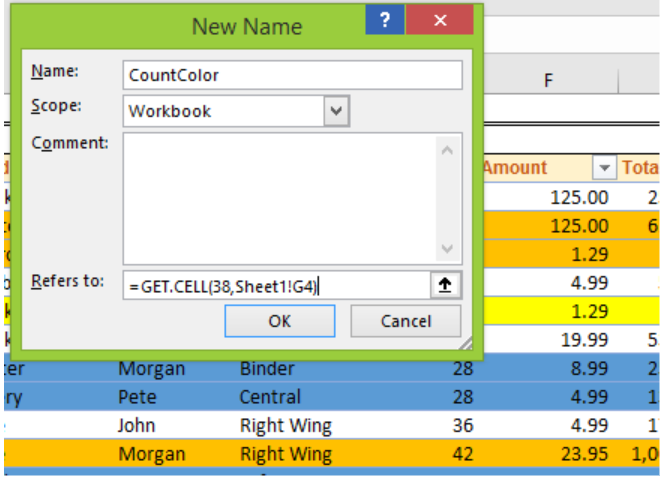Figure 8 – Count colors

• We will click on OK
• We will name Column H, as ColorCountFigure 9 – How to use the countif color function

• In Cell H4, we will enter the formula =CountColor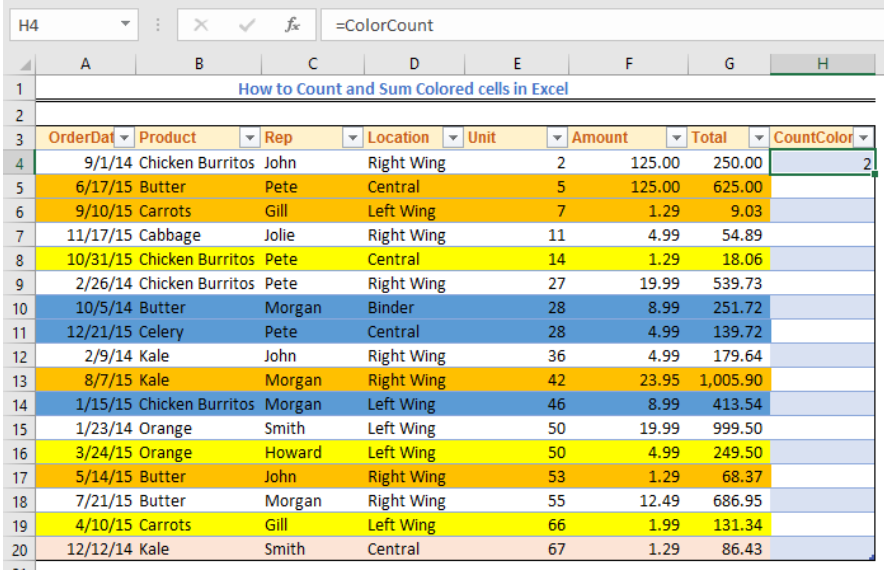Figure 10 – Using the count color process

• We will use the fill handle tool to enter other cells in that column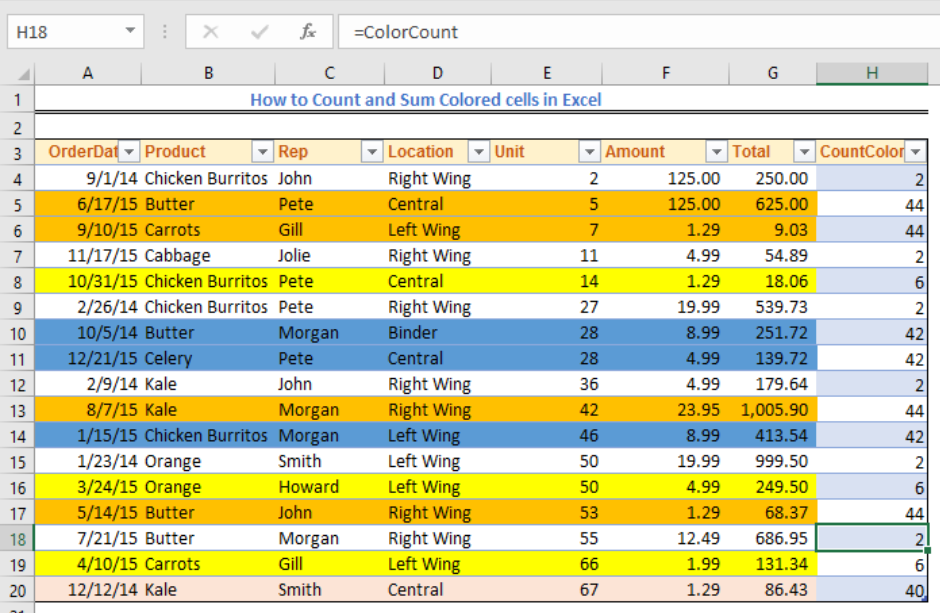Figure 11 – How to count colors

• Our formula will return 0 if there is no background color and a unique number if there is a color in the background
• From the number indicators, we will fill some cells with the colors we have in our table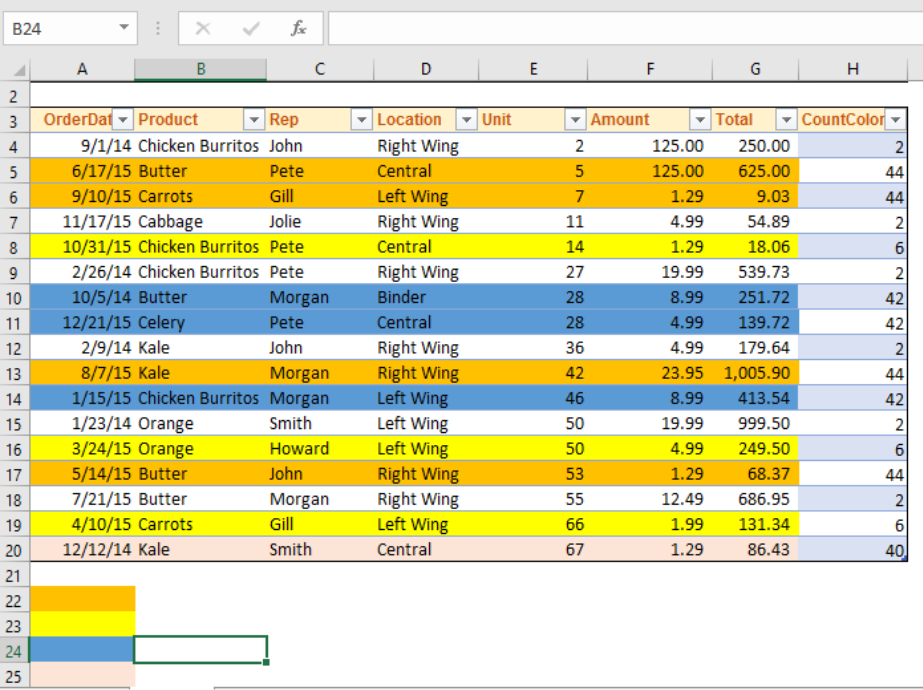Figure 12 – How to count highlighted cells

• In Cell B22, we will enter the formula below

`=COUNTIF(\$H:\$H,44)`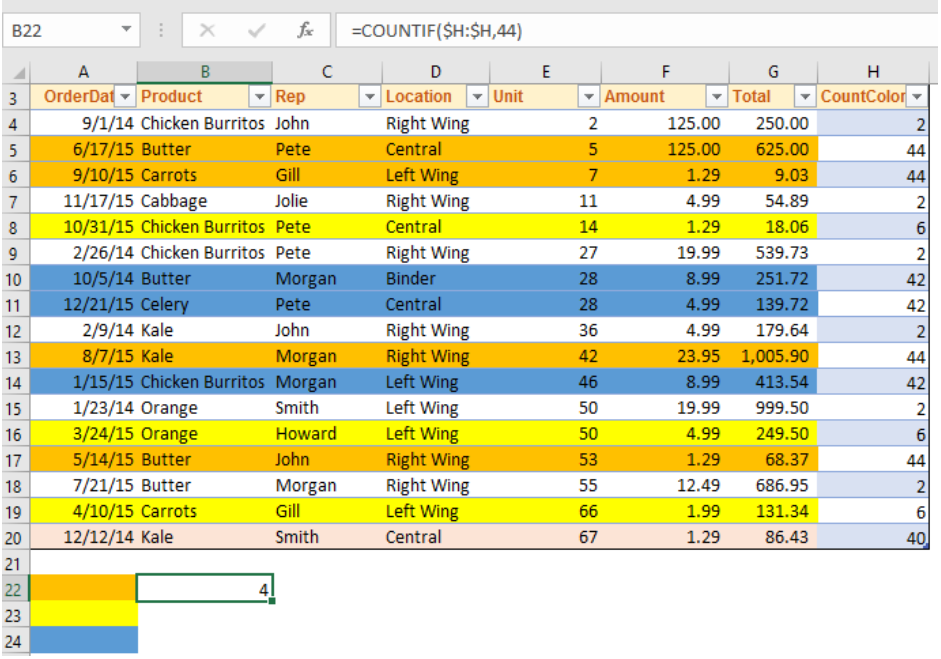Figure 13 – Count colors in the worksheet

• In Cell B23, we will enter this formula below

`=COUNTIF(\$H:\$H,6)`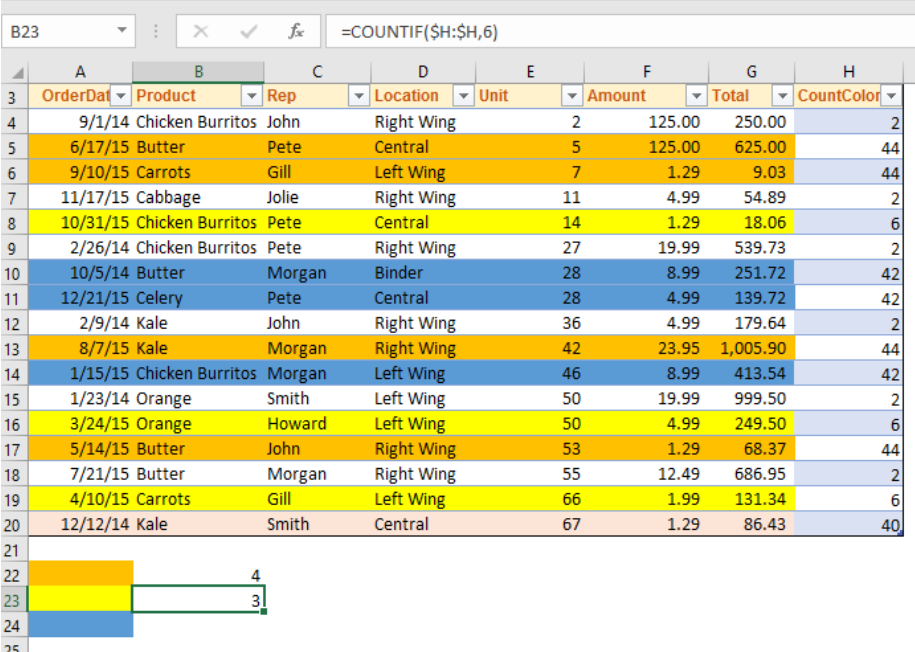Figure 14 – Using the fill handle tool to finish the countif color fill function

• In Cell B24, we will enter this formula below

`=COUNTIF(\$H:\$H,42)`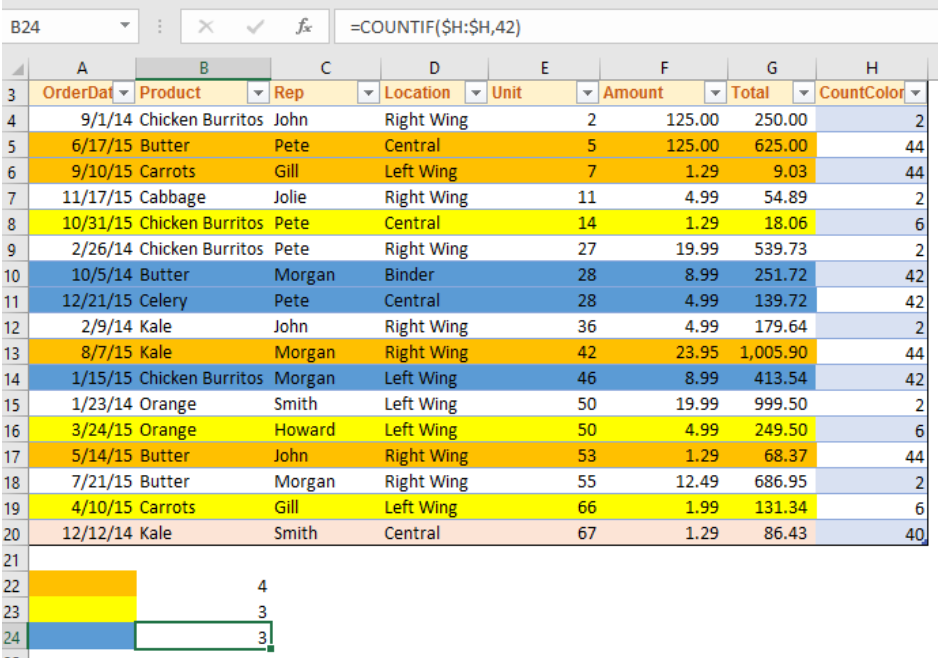Figure 15 – Using the fill handle tool to finish the countif color fill function

## How to Count Colored Cells using VBA

We can use a VBA macro code to count cells swiftly.

• We will go to the Developer Tab, navigate to the code group and select Visual Basic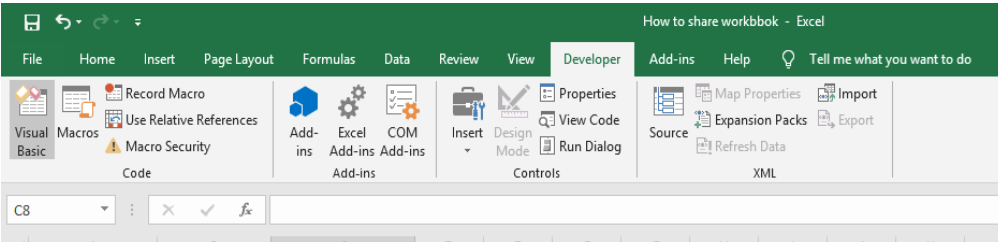Figure 16 – Using the vba display

• In the VB Editor, we will right-click on Microsoft Excel Objects and hover around insert
• Next, we will select Module from the drop-down menuFigure 17 – Macro box

• Now, we will copy and paste the VBA code below

`Function ColorCount(rColor As Range, rRange As Range)`

`    Dim rCell As Range`

`    Dim lCol As Long`

`    Dim vResult`

`    lCol = rColor.Interior.ColorIndex`

`    For Each rCell In rRange`

`        If rCell.Interior.ColorIndex = lCol Then`

`            vResult = 1 + vResult`

`        End If`

`    Next rCell`

`    ColorCount = vResult`

`End Function`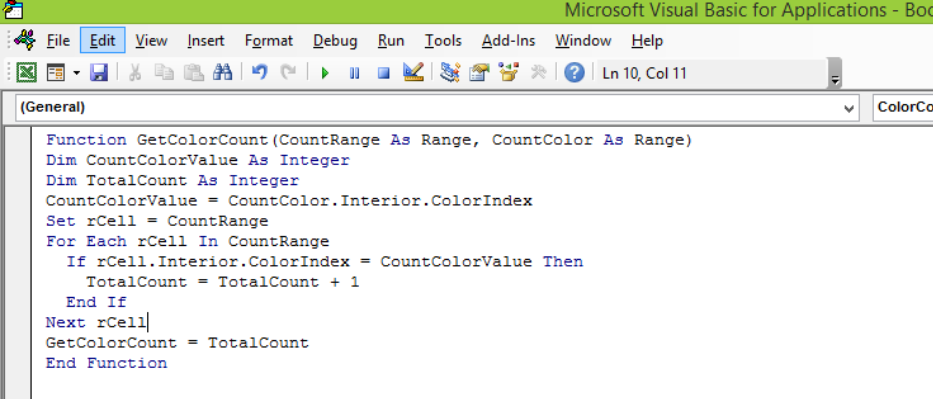Figure 18 – Using VBA to count colored cells

• We will save and close the VBA to go back to our Worksheet
• In the adjacent column (Column H), we will fill cells with our desired colors and enter the formula below

`=GetColorCount(\$A\$A4:\$A\$A20,A10)`

Where A10 represents the specific color we want to count and will change with when we have any color change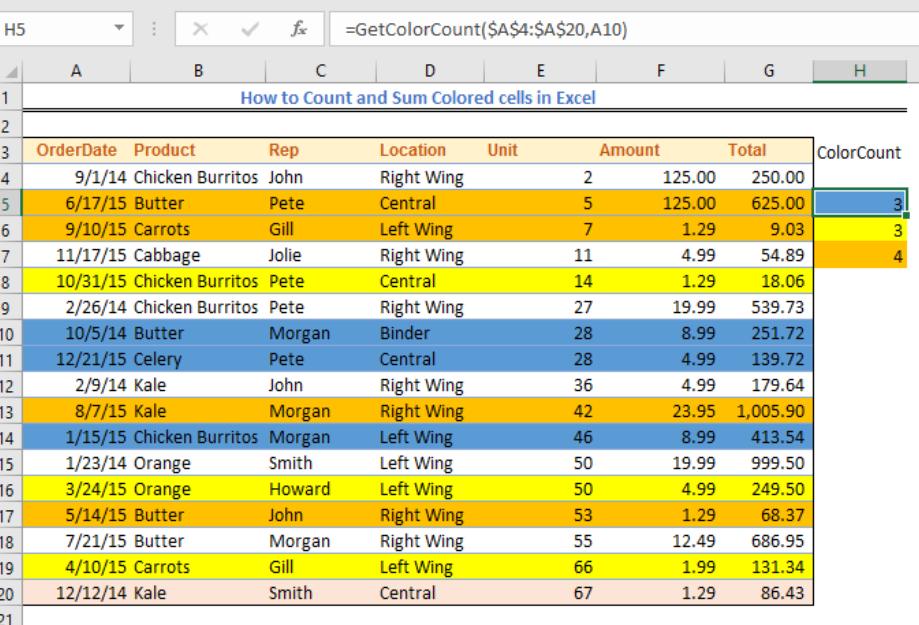Figure 19 – How to use Get Color Count function

## Instant Connection to an Excel Expert

Most of the time, the problem you will need to solve will be more complex than a simple application of a formula or function. If you want to save hours of research and frustration, try our live Excelchat service! Our Excel Experts are available 24/7 to answer any Excel question you may have. We guarantee a connection within 30 seconds and a customized solution within 20 minutes.